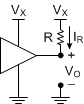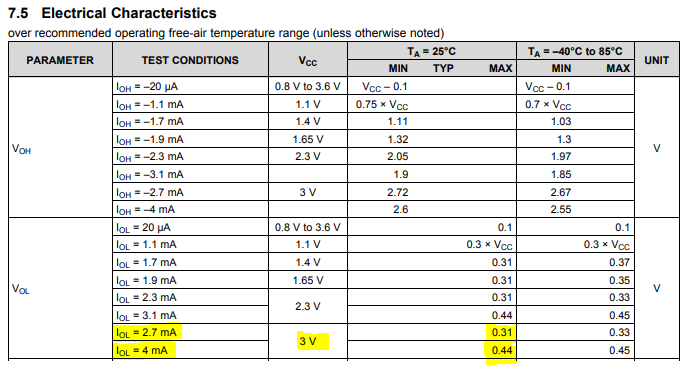If you have a related question, please click the "Ask a related question" button in the top right corner. The newly created question will be automatically linked to this question.

# [FAQ] How do I size pull-up or pull-down resistors?

Other Parts Discussed in Thread: SN74AUP1G34

FAQ: Logic and Voltage Translation > Input Parameters >> Current FAQ

Pull-up and pull-down resistors are required in many logic systems to provide a valid logic state when a wire connected to a CMOS input is unused.

The short answer is, if you aren't sure, use a 10kΩ resistor and adjust if you have problems.  The long answer follows.

There are two main areas of concern:

(1) Current

(a) The capability of your driver to source or sink the required current

(b) Leakage can cause a voltage drop across large resistors

(2) Transition rate - most CMOS inputs require a minimum input transition rate, and the resistor chosen will impact this.

(1) Drive Current

The governing equation for voltage across a resistor is called Ohm's Law, most commonly given as V = I * R

For an example, let's connect a resistor from a 3-state buffer's output to Vcc, commonly known as a 'pull-up resistor'When the buffer's output is active, Vo is controlled by the buffer.

If the output is driving HIGH, then Vo = Vx, and the voltage drop across R is Vx - Vx = 0 V, making IR = 0.

If the output switches to driving LOW, we first assume that Vo = 0 V, and now IR = (Vx - 0) / R

From the datasheet of the driver, we can determine the best value for R given this information.  Here's a snapshot from the SN74AUP1G34 datasheet:Choosing a supply value of 3V and knowing that the output voltage will be ~0.31 V at 2.7 mA drive current, I can adjust my above equation to this:  2.7 mA = (3V - 0.31V) / R

Rearranging and solving for R yields:  R ~= 996 Ω, or R = 1 kΩ for an easier value.

This is not the only value of resistor that would work -- in theory, we could use any value larger than this as well, since the current would be reduced.  So why don't we just use a 100 MΩ resistor in every circumstance to save power?

One reason is leakage current. When the output of the buffer is in the high-impedance mode, it will have some small amount of leakage current, and that will produce a voltage drop across the pull-up resistor (V = I*R). If the resistor is large, this voltage drop can be very significant.

Another reason is the delay that is cause, which is covered in the next section.

(2) RC Time Constant

An RC circuit will take time to charge.  The whole purpose of a pull-up resistor is to hold a line at a valid level while it is not in use, so it would defeat the purpose if it took a really long time for the line to reach said valid level.

The amount of time can be quickly estimated using t = 3*R*C.

You might be asking "where does this C come from" -- this is the parasitic capacitance on the line.  We typically assume 15 pF if it isn't given, but you can determine this for your system based on what's connected to the line, and the size of the trace/type of the transmission line.

Assuming you want a pretty fast response, it's a good idea to keep R relatively low. Most CMOS devices will have a limitation on the input transition rate (usually in the ~20ns/V range), which can affect the requirements for pull-up resistor.